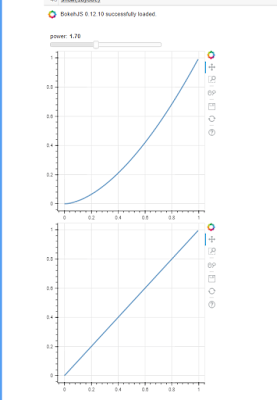# How to make one slider control two figures?

Using Jupyter notebook, how to have one slider interactively update two functions in two figures?

Using Bokeh Javascript Callback slider power example, I tried adding a second set of variables x and y but keep using the same callback. The graphs do not update anymore. Any suggestions? I also was trying to do the same with Pywidget but did not get anywhere. The end goal is to have a dashboard with an input composed of multiple slider, and an output composed of multiple plots all dependent on the same set of sliders input.

from bokeh.layouts import column

from bokeh.models import CustomJS, ColumnDataSource, Slider

from bokeh.plotting import Figure, output_notebook, show

output_notebook()

x = [x*0.005 for x in range(0, 200)]

y = x

x1 = [x1*0.005 for x1 in range(0, 200)]

y1 = x1

source = ColumnDataSource(data=dict(x=x, y=y))

source1 = ColumnDataSource(data=dict(x1=x1,y1=y1))

plot = Figure(plot_width=400, plot_height=400)

plot.line(‘x’, ‘y’, source=source, line_width=3, line_alpha=0.6)

plot1 = Figure(plot_width=400, plot_height=400)

plot1.line(‘x1’, ‘y1’, source=source1, line_width=3, line_alpha=0.6)

callback = CustomJS(args=dict(source=source, source1=source1), code="""

var data = source.data;

var data1 = source1.data;

var f1 =cb_obj.value

var f = cb_obj.value

x = data[‘x’]

y = data[‘y’]

x1 = data[‘x1’]

y1 = data[‘y1’]

for (i = 0; i < x.length; i++) {

y[i] = Math.pow(x[i], f)

}

for (i = 0; i < x1.length; i++) {

y1[i] = Math.pow(x1[i], f1)

}

source.change.emit();

source1.change.emit();

“”")

slider = Slider(start=0.1, end=4, value=1, step=.1, title=“power”)

slider.js_on_change(‘value’, callback)

layout = column(slider, plot,plot1)

show(layout)

``

Output is shown in attached imageHi Ali,

Regards,

Eugene

···

On Thursday, November 9, 2017 at 7:35:09 PM UTC+7, Ali Tarraf wrote:

Using Jupyter notebook, how to have one slider interactively update two functions in two figures?

Using Bokeh Javascript Callback slider power example, I tried adding a second set of variables x and y but keep using the same callback. The graphs do not update anymore. Any suggestions? I also was trying to do the same with Pywidget but did not get anywhere. The end goal is to have a dashboard with an input composed of multiple slider, and an output composed of multiple plots all dependent on the same set of sliders input.

from bokeh.layouts import column

from bokeh.models import CustomJS, ColumnDataSource, Slider

from bokeh.plotting import Figure, output_notebook, show

output_notebook()

x = [x*0.005 for x in range(0, 200)]

y = x

x1 = [x1*0.005 for x1 in range(0, 200)]

y1 = x1

source = ColumnDataSource(data=dict(x=x, y=y))

source1 = ColumnDataSource(data=dict(x1=x1,y1=y1))

plot = Figure(plot_width=400, plot_height=400)

plot.line(‘x’, ‘y’, source=source, line_width=3, line_alpha=0.6)

plot1 = Figure(plot_width=400, plot_height=400)

plot1.line(‘x1’, ‘y1’, source=source1, line_width=3, line_alpha=0.6)

callback = CustomJS(args=dict(source=source, source1=source1), code="""

var data = source.data;

var data1 = source1.data;

var f1 =cb_obj.value

var f = cb_obj.value

x = data[‘x’]

y = data[‘y’]

x1 = data[‘x1’]

y1 = data[‘y1’]

for (i = 0; i < x.length; i++) {

y[i] = Math.pow(x[i], f)

}

for (i = 0; i < x1.length; i++) {

y1[i] = Math.pow(x1[i], f1)

}

source.change.emit();

source1.change.emit();

“”")

slider = Slider(start=0.1, end=4, value=1, step=.1, title=“power”)

slider.js_on_change(‘value’, callback)

layout = column(slider, plot,plot1)

show(layout)

``

Output is shown in attached image